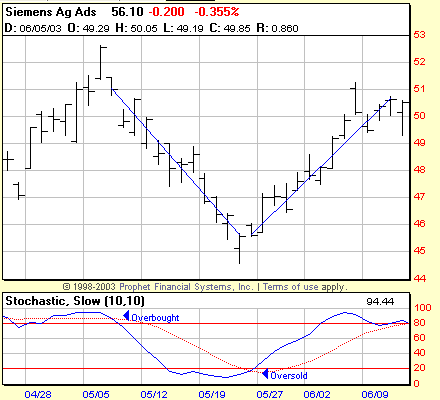# Stochastics

This classic momentum indicator was first popularized by George Lane.

Stochastics are based on the observation that in an uptrend as prices increase, closing prices gravitate to the upper end of the price range. In downtrends as prices fall you expect prices to close at the lower end of the range.

The stochastics indicator compares closing price to it’s price range over a given time period.

Fourteen is the standard time period setting for this indicator.Two lines are used: %K and %D

The formula for %K is:

%K= 100[(C – L14)]/ (H14 – L14)]

C = latest close

L14 = the lowest close for the past fourteen periods

H14 = the highest high for the past fourteen periods

The formula shows where the closing price is in relation to the price range over a given period (14 days in the example above) and plots it on a scale of 0 to 100.

The %K line uses the difference between todays close and the lowest low of the past 14 days, and divides that by the largest high-low range of the past 14 days. This ratio is multiplied by 100 to produce an oscillator that moves between 0 and 100. Like RSI, readings above 70 and below 30 are considered extreme.

A high reading above 80 shows that the price is currently near the top of the range for the period, and a reading below 20 shows that the price is currently near the bottom of the range for the period.

%K shows you the momentum of the price relative to the range.

The %D Line

The %D line is more important and produces the major signals. %D is a 3 period moving average of the %K line – it is the high to low ratio oscillator. This formula produces the version called fast stochastics.

By taking another 3 period moving average of %D a smoother version called slow stochastics is created. Signals from slow stochastics are more reliable and popular in use.

These formulas create 2 lines that oscillate between 0 and 100 on a vertical scale.

Interpretation of Stochastics

The most important signal to watch for is a divergence between the D line and price when D is an extreme area such as above 80 or below 20.

Buy signals are generated when the faster k line crosses above the D line, and sell signals are generated when the k line crosses below the D line. These crossovers are equivalent to the zero line crossovers in the other oscillators.

RSI and Stochasics

RSI is a good indicator to use alongside stochastics. The RSI line is less volatile than stochastics – look for the RSI to confirm overbought/oversold signals given by stochastics.